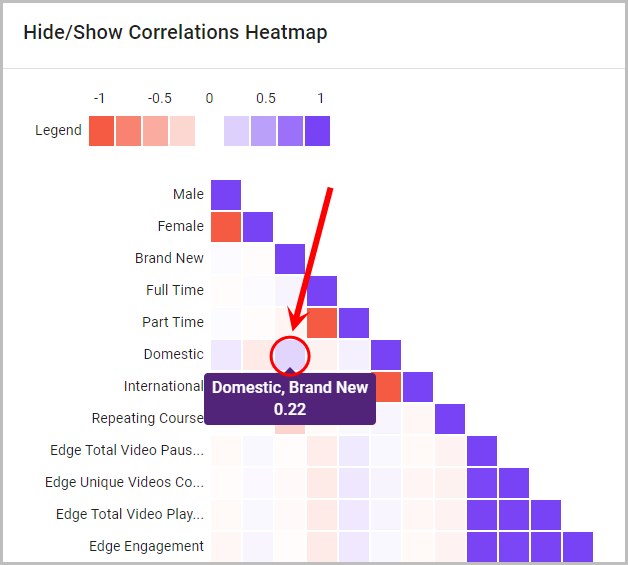## Overview

The Correlations section measures the linear relationship between two quantitative variables (e.g. Mid-Semester Exam and Video Engagement).

### What is a Correlation?

A Correlation is defined as a measure of the linear relationship between two quantitative variables (e.g. Mid Semester Exam and Video Engagement). The Pearson Correlation Coefficient has been used in the heatmap displayed below which results in values between -1 and 1.

• 0 means that the two variables are not correlated (i.e. No relationship between the variables at all).
• A correlation greater than 0 indicates a positive correlation. A positive correlation occurs when one variable moves higher or lower and the other variable moves in the same direction with the same magnitude.
• A correlation less than 0 indicates a negative correlation. A negative correlation indicates that both variables move in the opposite direction.

## Correlation Summary

The correlation summary table displays a list of correlated variables and their correlation coefficient, which is a measure that calculates the strength of the relationship between the relative movements of the two variables.

Click on the Correlation Coefficient column title to sort the table by the highest or lowest correlation coefficient value.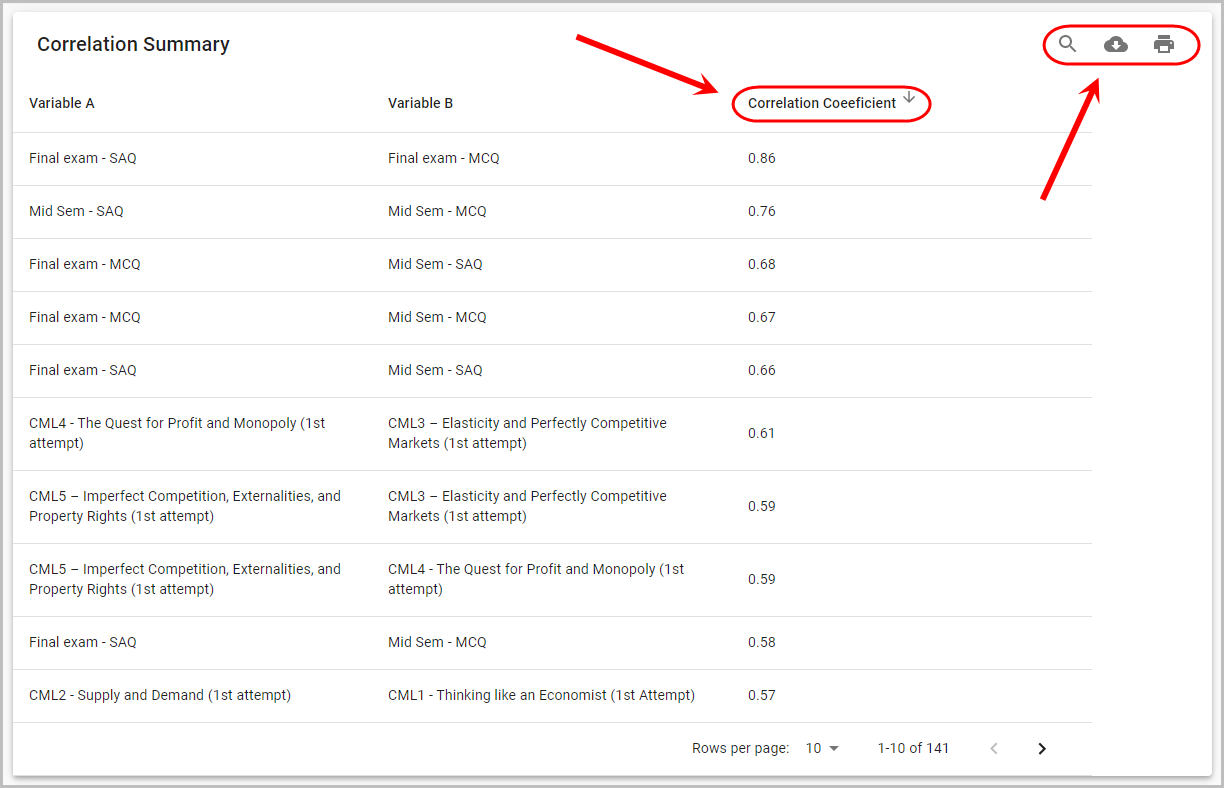## Correlations Scatter Plot

1. Select two different variables from the dropdown lists to compare them on the scatter plot.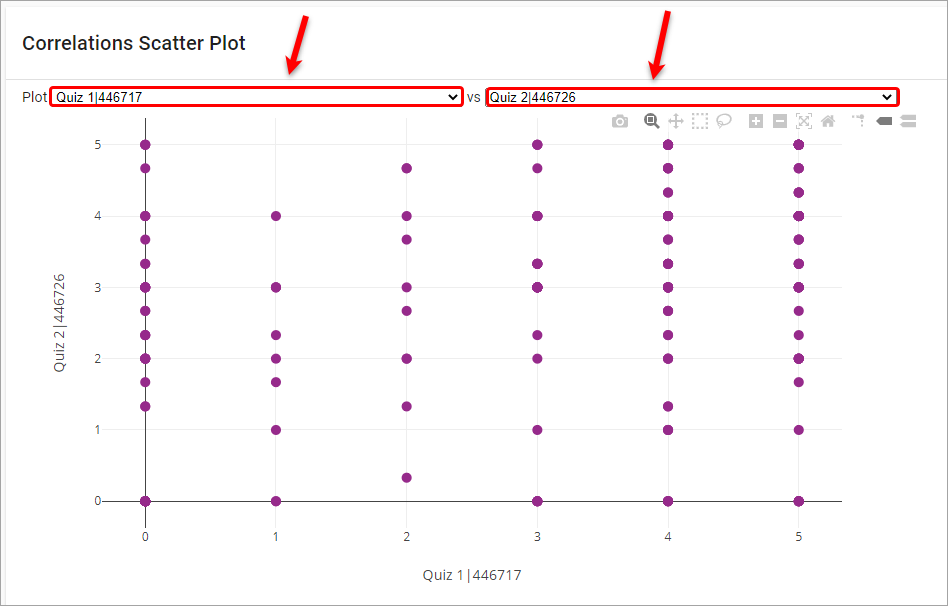1. Use the navigation tools to obtain your desired view of the scatter plot results.
2. Click on the camera button to download the current scatter plot view as a PNG file.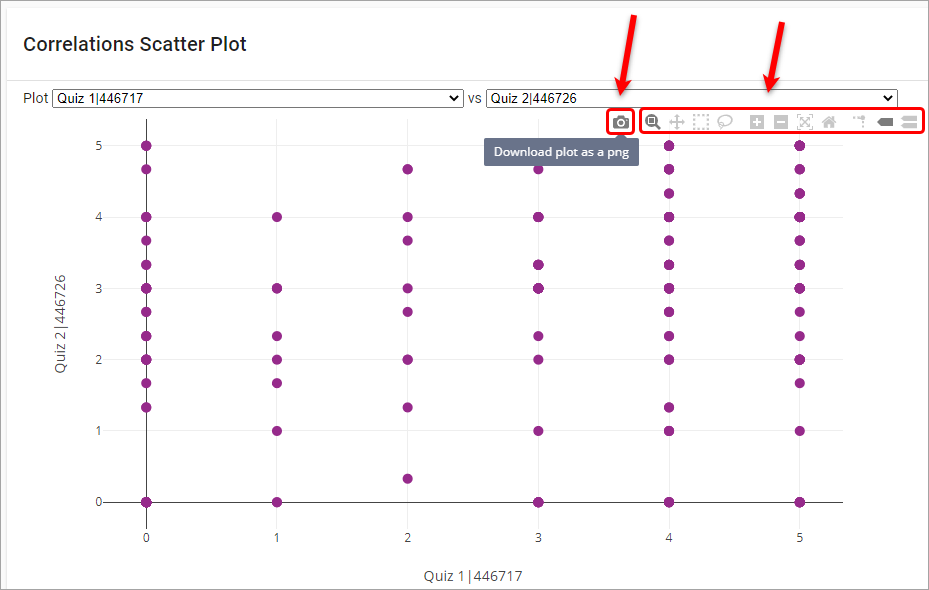## Correlations Heatmap

The following guidelines will assist in interpreting the heat map: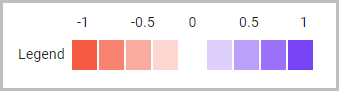• A correlation less than 0 indicates a negative correlation between two variables.
• A correlation of 0 indicates no relationship between two variables.
• A correlation greater than 0 indicates a positive correlation between two variables.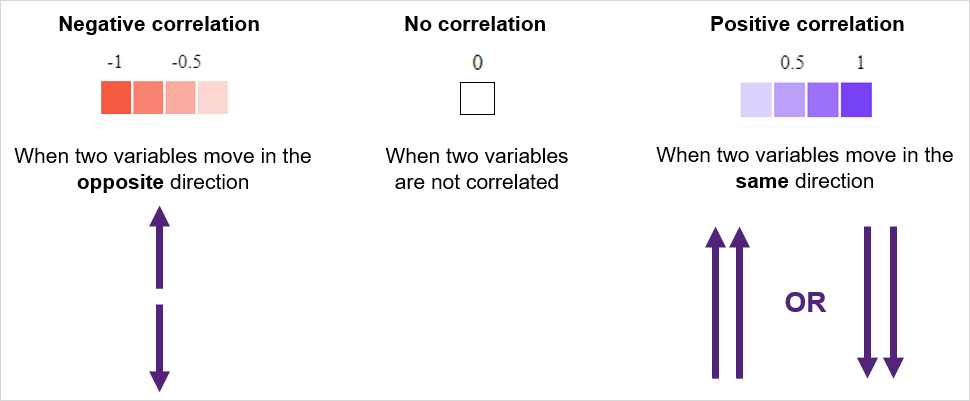### Expand the heatmap section

Click on the Hide/Show Correlations Heatmap section to view the heatmap.

### Compare variables

Hover your mouse over a particular square to view the correlation coefficient of the two variables.